﻿ 20-sim webhelp > Toolboxes > Mechatronics Toolbox > Servo Motor Editor > Theory > Basic Principles > Brushless DC Motors

# Brushless DC Motors

Navigation:  Toolboxes > Mechatronics Toolbox > Servo Motor Editor > Theory > Basic Principles >

# Brushless DC MotorsGiven motor with three coils, where the coils are mounted in the stator with a spatial displacement of 120°. The coils are connected in a star-formation as shown in the figure below.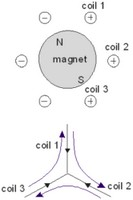In Brushless DC motors, the coils and magnets are designed so that a trapezoidal torque function Kc(q) is found as shown below: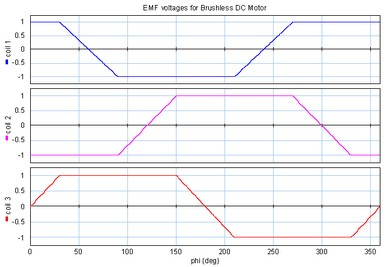To get a constant torque each coil current is a block wave as shown in the figure below. Although the current is thus alternating, the term DC is used to distinguish this type of motor from motors that used a sinusoidal current. The block wave current is provided through "electronic commutation". The current is generated by a three phase amplifier that gets the exact switching points from a motor controller.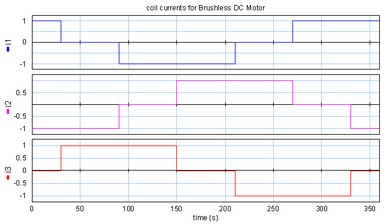As can easily be seen from the graphs, the resulting torque is constant and twice the current times the amplitude of the coil function:To get an equivalent of the coil model we can write this equation as: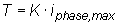We have to realize that here i is not a constant current anymore but the maximum coil current! Because the coil current is the current of one of the three phases, in literature i is mostly written as the maximum phase current. It is the maximum current that can be measured at each of the three terminals of the motor.

In a derivation that goes beyond the scope of this introduction (see Compter, 2004), it can be shown that the electromotive force can be found as: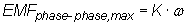Here the electromotive force is defined as the maximum induced voltage between two phases. The resulting motor model is shown below: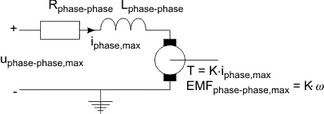The voltage of the motor model is commonly known as the maximum phase to phase voltage. It is the maximum voltage that can be measured between any pair of two terminals. The phase to phase resistance and phase to phase inductance are defined equivalent as the resistance and inductance that can be measured between any pair of two terminals.

## Limits

Brushless DC motors have no mechanical commutation, and therefore do not suffer from the limits caused by mechanical commutation. Consequently Brushless DC motors can run at much higher speeds and are not limited by a maximum voltage. The speed of Brushless DC motors is only limited by the bearings.

Just like brush DC motors the magnets impose an upper limit for the current and torque in a Brushless DC motor.